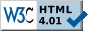Shop Amazon Warehouse Deals - Deep Discounts on Open-box and Used ProductsApril
Sun Mon Tue Wed Thu Fri Sat

29

2006
Months
Apr

### Sat, Apr 29, 2006 1:24 pm

PHP - Exec

The PHP `exec` function can be used to call external programs. For instance, if I wish to create a webpage that displays the MD5 checksum for a file, I can call the md5sum program that is present on Unix and Linux systems. If I called the program from a shell prompt on the system, I would see something like the following:

``` # md5sum file.txt 529dc67dde9486a1af8353915ab94870 file.txt ```

Using PHP, I can get the MD5 checksum with the following code:

``` <?php \$filename="mboxgrep-0.7.9-1.i386.rpm"; \$md5sum = exec('md5sum '.\$filename); \$md5sum = substr(\$md5sum,0,strpos(\$md5sum,' ')); ?> ```

The results of the call to the external `md5sum` program are stored in a variable named `md5sum`. The `md5sum` program returns the MD5 checksum followed by a space and then the filename. The filename can be stripped away by using `strpos` to determine the position of the space in the string and then `substr` can be used to remove all of the charcters from the string starting with the space to the end of the string.

Since I need to calculate the MD5 checksum, aka hash, regularly, I can create a function that calls the external md5sum program to do so.

``````
function md5sum(\$filename) {

\$hash = exec('md5sum '.\$filename);
// The md5sum command returns the MD5 hash followed by a space and the
// filename. Remove the space and filename.
\$hash = substr(\$hash,0,strpos(\$hash,' '));
return(\$hash);

}
```
```

But what if you call an external program that returns multi-line output. If you just store the results obtained by using `exec` to call the program, you will get only the last line of output for the program.

For instance, I can use the command `rpm -qp --requires file.rpm` to determine what other software is required by a RPM file. If I call that program with PHP's `exec` function and assign the results to a variable, `requires`, however, I get just the last line of the results of calling `rpm -qp --requires`, which produces multiline output.

``` <?php \$filename="mboxgrep-0.7.9-1.i386.rpm"; \$requires = exec('rpm -qp --requires '.\$filename); ?> ```

What I need to do instead, is put the output of the external command into an array. When using the `exec` function, I can specify an array to be used to hold the output, by putting a comma after the command to be called and then specifying an array to hold the output of the command.

``` <?php exec(external_command, \$output_array); ?> ```

For instance, to obtain the output from the rpm command above, I could use the following code:

``````
<?php

\$filename="mboxgrep-0.7.9-1.i386.rpm";
exec('rpm -qp --requires '.\$filename, \$requires);

for (\$i = 0; \$i < count(\$requires); \$i++) {
print "\$requires[\$i]<br>\n";
}

?>
```
```
The `exec` function is used to call the program, storing the output from the `rpm` command in the array `\$requires`. I can then use a `for` loop to print each of the lines in the array, putting a `<br>` tag at the end of each line, so that the HTML output is more readable and matches that of the program. I also use `/n` to create a new line at the end of each line of output so the source HTML code is more readable, also.

References:Shop Amazon Local - Subscribe to Deals in Your Neighborhood